## Electrostatics Exam3 and Problem Solutions

Electrostatics Exam3 and  Problem Solutions

1. If we first touch A to B then A to C, find the final charges of these spheres. (charges of spheres;

1st sphere:8q, 2nd sphere:-2q, 3rd sphere:-14q)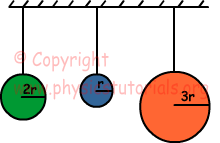qA+qB=8q-2q=6q

qr=qtotal/rtotal=6q/(2r+r)=2q/r

Charge of qA'=2r.2q/r=4q

Charge of qB=r.2q/2=2q

qtotal=qA'+qC=4q-14q=-10q

qr=qtotal/rtotal=-10q/(3r+2r)=-2q/r

Final charges of spheres:

qAfinal=2r.(-2q)/r=-4q

qCfinal=3r.(-2q)/r=-6q

qB=r.2q/2=2q

2. Look at the picture given below.Find the tension in the rope.Free body diagram of the system is given below;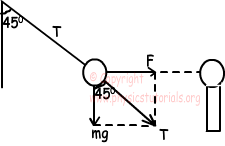tan450=F/mg=1

F=mg

k.q.q/d2=m.g

q=d√mg/k

We find T using pythagorean theorem

T2=F2+mg2

T2=(mg)2+(mg)2

T=mg√2

3. A, B and C spheres are charged. If A repels B and attracts C, and B is positively charged, find the types of charges of each sphere.

If A repels B, then they must be charged with same charge; and if A attracts C then they must be charged with opposite charges.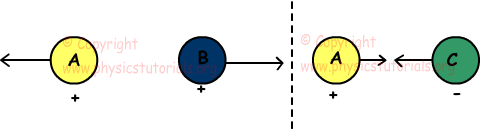4. We put negatively charged rod closer to neutral electroscope. If we ground electroscope, what will be the motion of the leaves of electroscope?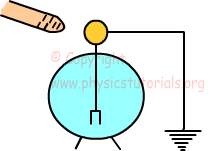When we put negatively charged rod near the sphere of electroscope, sphere is positively charged by induction and negative charges are repelled to the leaves. When we ground electroscope, negative charges move to the ground and leaves becomes neutral and closed.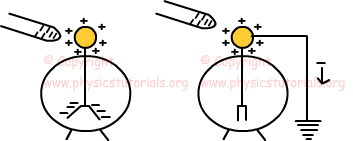5. Find charge of q3 to make total force acting on q2 zero.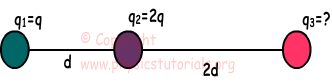F12 must be equal Fub23

k.q1.q2/d2=k.q2.q3/(2d)2

q1/d2=q3/4d2

q3=4q1=4q

Author: